# Introduction to Processing Digital Sounds part 4 Barb

• Slides: 15
Download presentation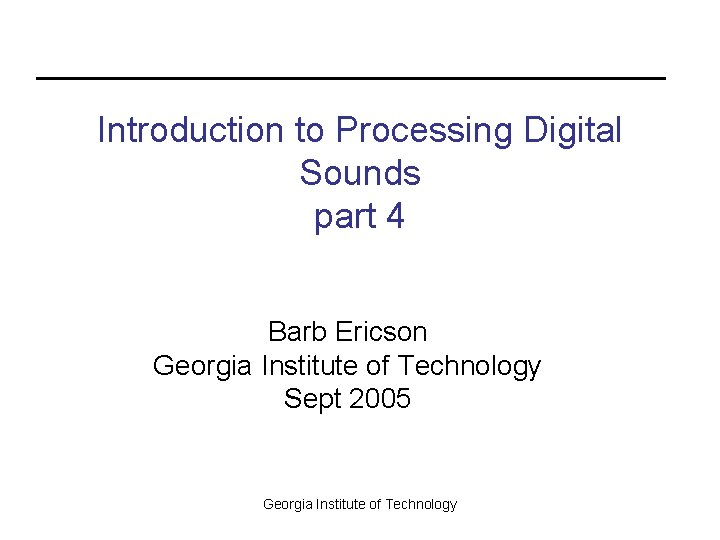Introduction to Processing Digital Sounds part 4 Barb Ericson Georgia Institute of Technology Sept 2005 Georgia Institute of Technology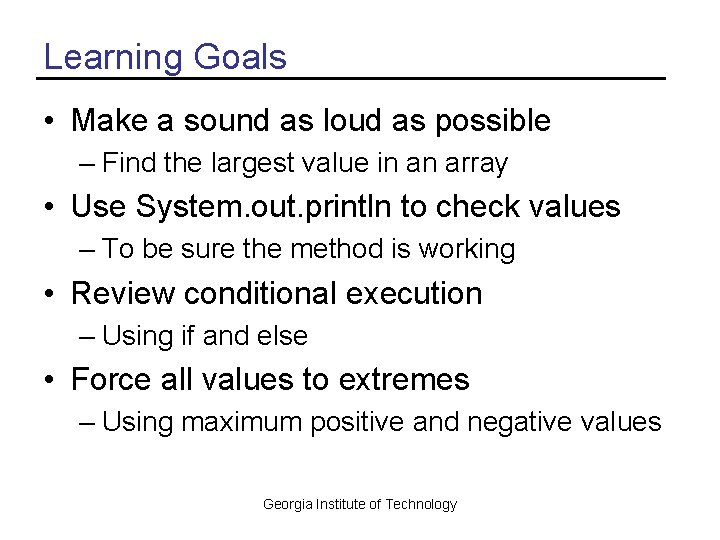Learning Goals • Make a sound as loud as possible – Find the largest value in an array • Use System. out. println to check values – To be sure the method is working • Review conditional execution – Using if and else • Force all values to extremes – Using maximum positive and negative values Georgia Institute of TechnologyNormalize Sounds • Make the whole sound as loud as possible – How loud can it be? • The max positive value is 32767. 0 • The max negative value is -32768. 0 – First we need to find the largest value (positive or negative) in the current sound • Create a variable to hold the max – And set it to the first value in the array • And loop through the array and if the absolute value of the current value is greater – Store that one instead Georgia Institute of Technology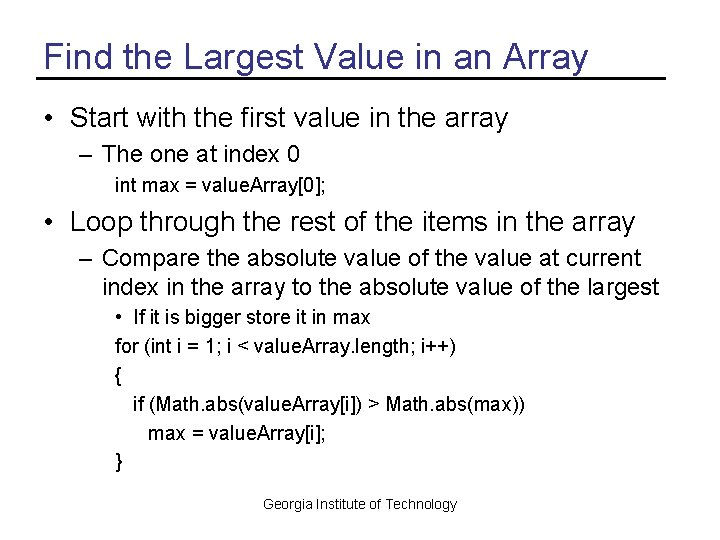Find the Largest Value in an Array • Start with the first value in the array – The one at index 0 int max = value. Array; • Loop through the rest of the items in the array – Compare the absolute value of the value at current index in the array to the absolute value of the largest • If it is bigger store it in max for (int i = 1; i < value. Array. length; i++) { if (Math. abs(value. Array[i]) > Math. abs(max)) max = value. Array[i]; } Georgia Institute of TechnologyFind the Largest Value in an Array • int max = first element 2000 | 1000 | -2344 | 100 | 3300 max = value. Array = 2000; for (int i = 1; i < value. Array. length; i++) { if (Math. abs(value. Array[i]) > Math. abs(max) max = value. Array[i]; } Georgia Institute of Technology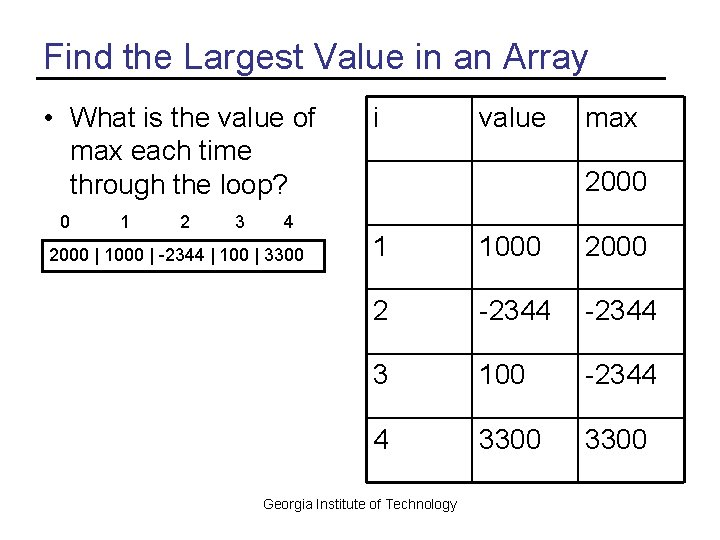Find the Largest Value in an Array • What is the value of max each time through the loop? 0 1 2 3 4 2000 | 1000 | -2344 | 100 | 3300 i value max 2000 1 1000 2 -2344 3 100 -2344 4 3300 Georgia Institute of Technology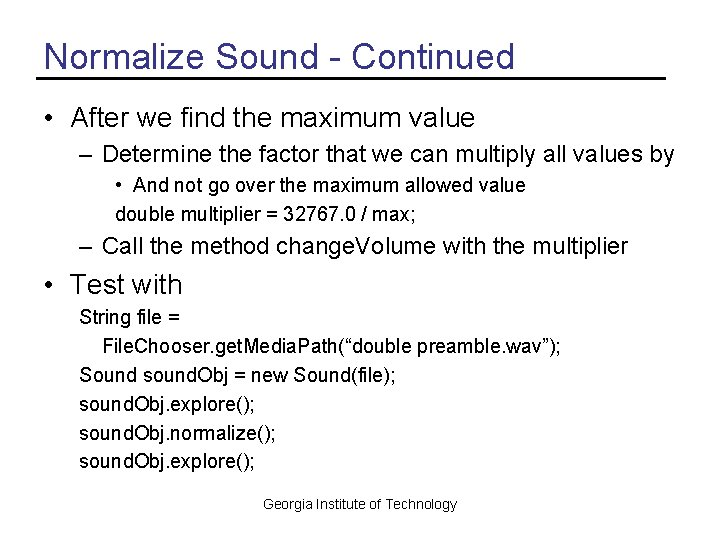Normalize Sound - Continued • After we find the maximum value – Determine the factor that we can multiply all values by • And not go over the maximum allowed value double multiplier = 32767. 0 / max; – Call the method change. Volume with the multiplier • Test with String file = File. Chooser. get. Media. Path(“double preamble. wav”); Sound sound. Obj = new Sound(file); sound. Obj. explore(); sound. Obj. normalize(); sound. Obj. explore(); Georgia Institute of TechnologyNormalize Method public void normalize() { Sound. Sample[] sample. Array = this. get. Samples(); Sound. Sample sample = sample. Array; int value = 0; int max = sound. Sample. get. Value(); // loop comparing values // to the current largest for (int i = 1; i < sample. Array. length; i++) { sample = sample. Array[i]; value = sample. get. Value(); if (Math. abs(value) > Math. abs(max)) { max = value; } } } // calculate the multiplier double multiplier = 32767. 0 / max; // change the volume this. change. Volume(multiplier); } Georgia Institute of TechnologyTesting Normalize • How do we know if it worked? – We can play the sound but it may not sound all that different – We can use the explorer to view the sound wave before and after we normalize the sound • And see if the values changed – Check more than one index – We can use System. out. println to print out the largest value and the index of it • We need to save the index of the maximum value • And use the explorer to check the value at that index Georgia Institute of Technology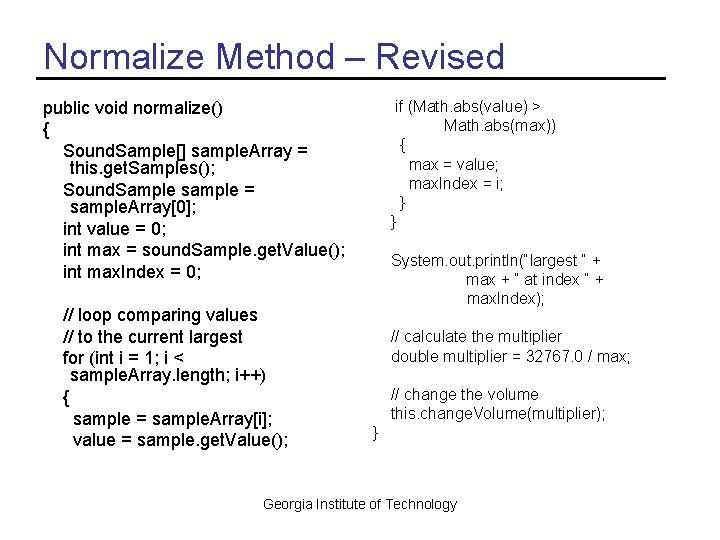Normalize Method – Revised public void normalize() { Sound. Sample[] sample. Array = this. get. Samples(); Sound. Sample sample = sample. Array; int value = 0; int max = sound. Sample. get. Value(); int max. Index = 0; // loop comparing values // to the current largest for (int i = 1; i < sample. Array. length; i++) { sample = sample. Array[i]; value = sample. get. Value(); if (Math. abs(value) > Math. abs(max)) { max = value; max. Index = i; } } System. out. println(“largest “ + max + “ at index “ + max. Index); // calculate the multiplier double multiplier = 32767. 0 / max; // change the volume this. change. Volume(multiplier); } Georgia Institute of Technology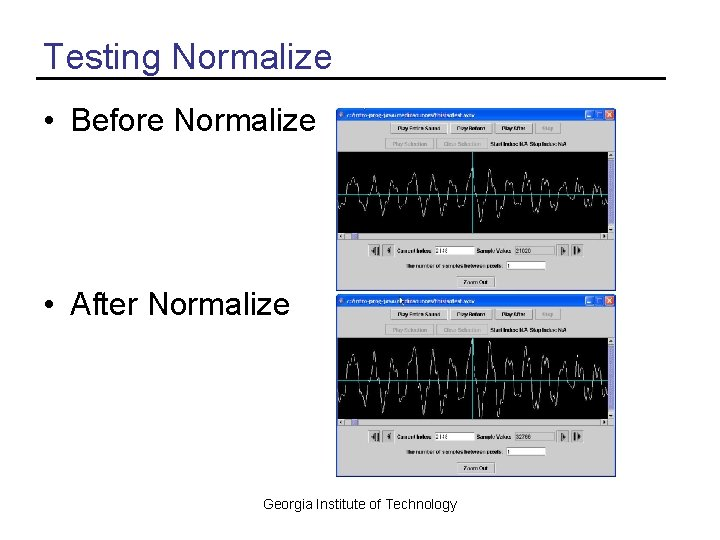Testing Normalize • Before Normalize • After Normalize Georgia Institute of TechnologyForce to Extremes • What if we want to make all values in a sound the maximum positive or negative value? – If the value is positive make it 32, 767 – If the value is negative make it -32, 768 • We need a way to execute code based on if a test is true – We can use a conditional (if and else) Georgia Institute of TechnologyConditionals • Allow you to only execute statements if an expression is true false if (expression) – Or (optionally) only if it is false • If you clean your room – You can go out else true Statement or block • Else – You must stay home statement Georgia Institute of Technology Statement or block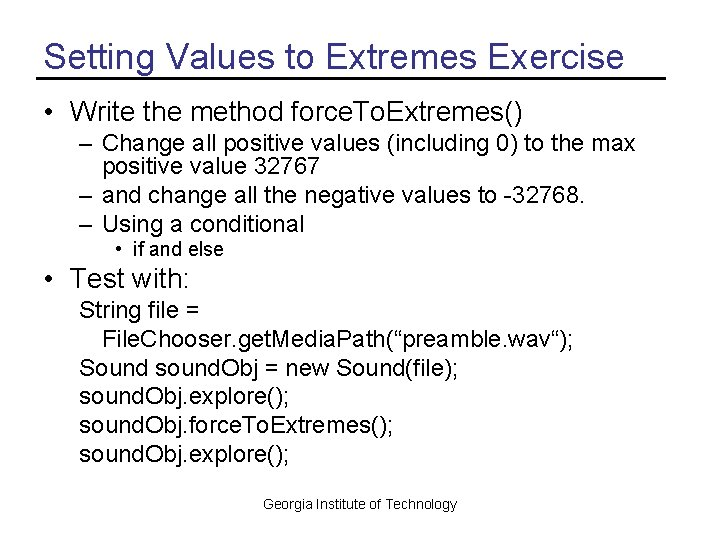Setting Values to Extremes Exercise • Write the method force. To. Extremes() – Change all positive values (including 0) to the max positive value 32767 – and change all the negative values to -32768. – Using a conditional • if and else • Test with: String file = File. Chooser. get. Media. Path(“preamble. wav“); Sound sound. Obj = new Sound(file); sound. Obj. explore(); sound. Obj. force. To. Extremes(); sound. Obj. explore(); Georgia Institute of Technology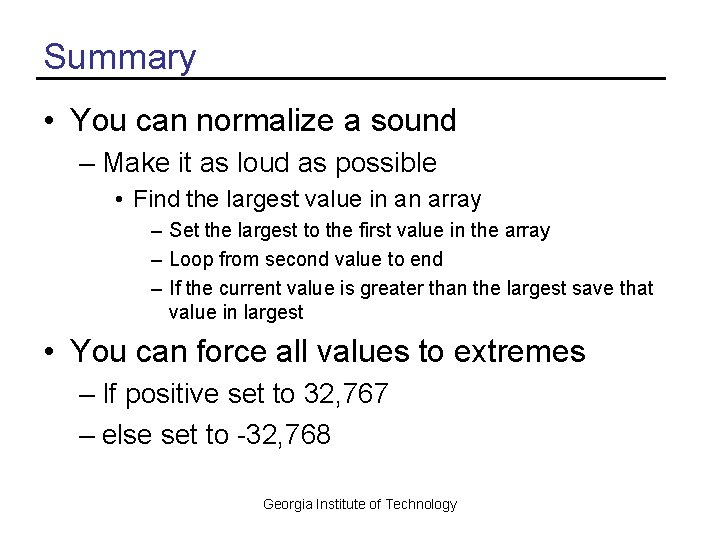Summary • You can normalize a sound – Make it as loud as possible • Find the largest value in an array – Set the largest to the first value in the array – Loop from second value to end – If the current value is greater than the largest save that value in largest • You can force all values to extremes – If positive set to 32, 767 – else set to -32, 768 Georgia Institute of Technology